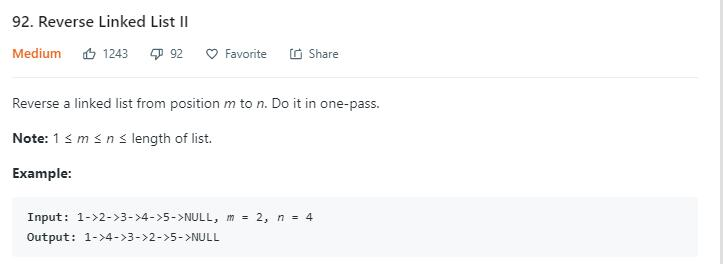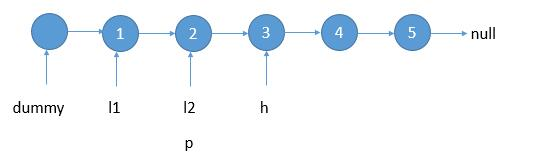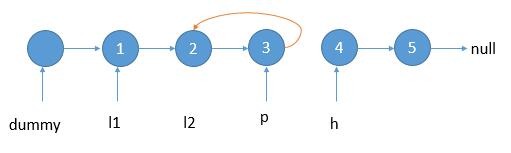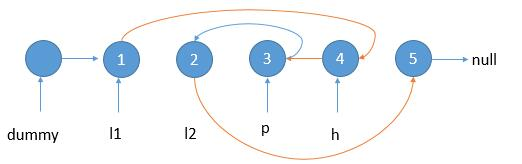# 题目描述（中等难度）# 解法一

m = 2, n = 4

1 2 3 4 5 加入哨兵节点 d，pre 简写 p，head 简写 h

0 1 2 3 4 5 往后遍历
^ ^
d h
p

0 1 2 3 4 5 此时 h 指向 m 的位置，记录 p 和 h 为 l1 和 l2
^ ^ ^
d p h

0  1  2 3 4 5 然后继续遍历
^  ^  ^
d  p  h
l1  l2

0  1  2  3 4 5 开始倒置链表，使得 h 指向 p
^  ^  ^  ^
d  l1 p  h
l2public ListNode reverseBetween(ListNode head, int m, int n) {
if (m == n) {
}
ListNode dummy = new ListNode(0);
int count = 0;
ListNode left1 = null;
ListNode left2 = null;
ListNode pre = dummy;
count++;
//到达 m，保存 l1 和 l2
if (count == m) {
left1 = pre;
}
// m 和 n 之间，倒转链表
if (count > m && count < n) {
continue;
}
//到达 n
if (count == n) {
break;
}
//两个指针后移
pre = pre.next;
}
return dummy.next;
}